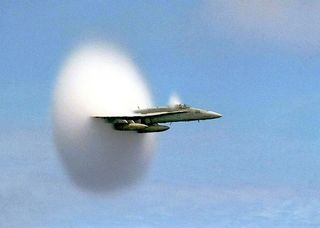# Force, Mass & Acceleration: Newton's Second Law of MotionIsaac Newton's First Law of Motion states, "A body at rest will remain at rest, and a body in motion will remain in motion unless it is acted upon by an external force." What, then, happens to a body when an external force is applied to it? That situation is described by Newton's Second Law of Motion.

According to NASA, this law states, "Force is equal to the change in momentum per change in time. For a constant mass, force equals mass times acceleration." This is written in mathematical form as F = ma

F is force, m is mass and a is acceleration. The math behind this is quite simple. If you double the force, you double the acceleration, but if you double the mass, you cut the acceleration in half.

Newton published his laws of motion in 1687, in his seminal work "Philosophiæ Naturalis Principia Mathematica" (Mathematical Principles of Natural Philosophy) in which he formalized the description of how massive bodies move under the influence of external forces.

Newton expanded upon the earlier work of Galileo Galilei, who developed the first accurate laws of motion for masses, according to Greg Bothun, a physics professor at the University of Oregon. Galileo's experiments showed that all bodies accelerate at the same rate regardless of size or mass. Newton also critiqued and expanded on the work of Rene Descartes, who also published a set of laws of nature in 1644, two years after Newton was born. Descartes' laws are very similar to Newton's first law of motion.

## Acceleration and velocity

Newton's second law says that when a constant force acts on a massive body, it causes it to accelerate, i.e., to change its velocity, at a constant rate. In the simplest case, a force applied to an object at rest causes it to accelerate in the direction of the force. However, if the object is already in motion, or if this situation is viewed from a moving inertial reference frame, that body might appear to speed up, slow down, or change direction depending on the direction of the force and the directions that the object and reference frame are moving relative to each other.

The bold letters F and a in the equation indicate that force and acceleration are vector quantities, which means they have both magnitude and direction. The force can be a single force or it can be the combination of more than one force. In this case, we would write the equation as ∑F = ma

The large Σ (the Greek letter sigma) represents the vector sum of all the forces, or the net force, acting on a body.

It is rather difficult to imagine applying a constant force to a body for an indefinite length of time. In most cases, forces can only be applied for a limited time, producing what is called impulse. For a massive body moving in an inertial reference frame without any other forces such as friction acting on it, a certain impulse will cause a certain change in its velocity. The body might speed up, slow down or change direction, after which, the body will continue moving at a new constant velocity (unless, of course, the impulse causes the body to stop).

There is one situation, however, in which we do encounter a constant force — the force due to gravitational acceleration, which causes massive bodies to exert a downward force on the Earth. In this case, the constant acceleration due to gravity is written as g, and Newton's Second Law becomes F = mg. Notice that in this case, F and g are not conventionally written as vectors, because they are always pointing in the same direction, down.

The product of mass times gravitational acceleration, mg, is known as weight, which is just another kind of force. Without gravity, a massive body has no weight, and without a massive body, gravity cannot produce a force. In order to overcome gravity and lift a massive body, you must produce an upward force ma that is greater than the downward gravitational force mg

## Newton's second law in action

Rockets traveling through space encompass all three of Newton's laws of motion.

If the rocket needs to slow down, speed up, or change direction, a force is used to give it a push, typically coming from the engine. The amount of the force and the location where it is providing the push can change either or both the speed (the magnitude part of acceleration) and direction.

Now that we know how a massive body in an inertial reference frame behaves when it subjected to an outside force, such as how the engines creating the push maneuver the rocket, what happens to the body that is exerting that force? That situation is described by Newton’s Third Law of Motion

Additional reporting by Rachel Ross, Live Science contributor.